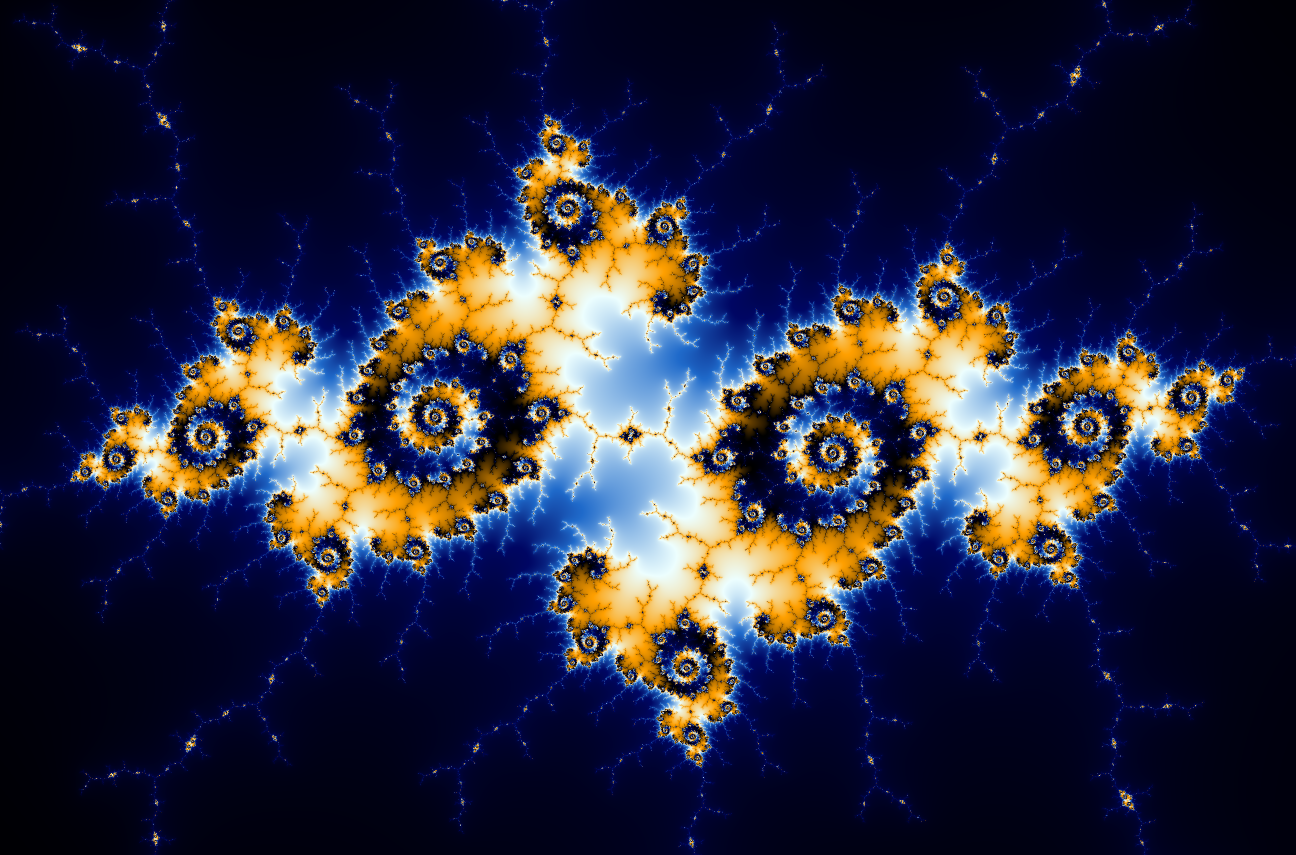"10. History is a branch of Mathematics. So is literature. Economics is a branch of religion."

- The Humans, p. 271

In The Humans, mathematics plays a starring role. The society that the protagonist hails from, Vonnadoria, worships mathematics. Professor Andrew Martin is also a mathematician who specialises in prime numbers. The book mentions several different mathematical theories, and on this page you can learn more about each of them.

## Riemann Hypothesis

"Bernhard Riemann, one of the extraordinary mathematical talents of the nineteenth century, formulated the problem in 1859. The hypothesis makes a very precise connection between two seemingly unrelated mathematical objects (namely, prime numbers and the zeros of analytic functions). If solved, it would give us profound insight into number theory and, in particular, the nature of prime numbers."

Borwein, Choi, Rooney, & Weirathmueller, 2007, p. 4

In The Humans Professor Andrew Martin draws extra-terrestrial attention for solving the Riemann hypothesis, which he described as "the most important unsolved problem in mathematics." (p. 59) The hypothesis (and fictional proof) show that the order of prime numbers is not random, but that they can in fact be predicted.

## Poincaré Conjecture

The Poincaré conjecture (a topological conundrum) had baffled the best minds on the planet for over 100 years and the solution could help us understand the shape of the universe. While working in an area of mathematics known as Algebraic Topology, Henri Poincaré attempted to determin the topological properties that characterised a sphere. Russian mathamatician Grigori Perelman solved the Poincaré conjecture quite recently. Yet Perelman avoided any fanfare and posted his solution online. You can read the three papers in which Perelman sets out his proof for yourself;

In the aftermath of publishing the solution Perelman turned down prize money of a million dollars and the Fields medal, which is considered to be the maths equivalent of a Nobel prize. He had all he could possibly want already, he said.

## Drake Equation

Devised by Frank D. Drake in 1961, the Drake equation was the first scientific attempt to estimate the number N of extra-terrestrial civilizations in the Galaxy, with which we might come in contact. Represented like this;

N = R x fp x ne x fl x fi x fc x L

• R represents the average rate of star formation per year in our galaxy,
• fp is the fraction of stars that host planetary systems,
• ne is the number of planets in each system that are potentially habitable,
• fl is the fraction of habitable planets where life originates and becomes complex,
• fi is the fraction of life-bearing planets that bear intelligence,
• fc is the fraction of intelligence bearing planets where technology can develop,
• and L is the mean lifetime of a technological civilization within the detection window.

However in The Humans Professor Andrew Martin suggests in one of his lectures that the Drake equation could be updated with extra fractions to include fcgas "Fraction who could give a shit about visiting or communicating with Earth," and fdsbthdr "Fraction who did so but the humans didn't realise." p. 157. Gladea, Balleta, and Bastiena (2012) provide a very good overview of the Drake equation in their research article "A stochastic process approach of the drake equation parameters."

Several other mathematics texts are mentioned in The Humans, such as The Book of Hexagonal Tessellation; Logarithmic Spirals and the Golden Mean, and The Zeta Function. As far as we can tell, these titles are fictional, however you can read some similar titles which we have listed below.